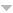## Publications

Export 45 results:
Sort by: Author Title Type [ Year]
2023
Ferrás, L., M. L. Morgado, and Magda Rebelo. "A generalised distributed-order Maxwell model." Mathematical Methods in the Applied Sciences 46 (2023): 368-387. Abstract

n/a

2022
Morgado, M. L., M. Rebelo, and L. L. Ferrás. "Finite Difference Schemes with Non-uniform Time Meshes for Distributed-Order Diffusion Equations." In Proceedings of the International Conference on Fractional Differentiation and its Applications (ICFDA’21), 239-244. Springer, 2022. Abstract
n/a
Faghih, Amin, and Magda Rebelo. "A spectral approach to non-linear weakly singular fractional integro-differential equations." Fractional Calculus and Applied Analysis (2022): 1-29. Abstract
n/a
2021
Ferrás, L., M. L. Morgado, and M. Rebelo. "A distributed order viscoelastic model for small deformations." In SYMCOMP 2021. Evora, Portugal, 2021.
Ribau, A. M., L. L. Ferrás, M. L. Morgado, M. Rebelo, M. A. Alves, F. T. Pinho, and A. M. Afonso. "A study on mixed electro-osmotic/pressure-driven microchannel flows of a generalised Phan-Thien–Tanner fluid." Journal of Engineering Mathematics 127 (2021).
Morgado, Lu{\'ısa M., Magda Rebelo, and Lu{\'ıs L. Ferrás. "Stable and convergent finite difference schemes on nonuniformtime meshes for distributed-order diffusion equations." Mathematics 9 (2021): 1975. Abstract
n/a
2020
Ribau, A. M., L. Ferrás, M. L. Morgado, M. Rebelo, and A. Afonso. "Analytical and numerical studies for slip ﬂows of a generalised Phan-Thien-Tanner ﬂuid." ZAMM Journal of applied mathematics and mechanics: Zeitschrift für angewandte Mathematik und Mechanik 100 (2020).
Morgado, M. L., and M. Rebelo. "Collocation Solution of Fractional Differential Equations in Piecewise Nonpolynomial Spaces." In Differential and Difference Equations with Applications., 2020.
Ferrás, L., N. Ford, M. L. Morgado, and M. Rebelo. "High-Order Methods for Systems of Fractional Ordinary Differential Equations and Their Application to Time-Fractional Diffusion Equations." Mathematics in Computer Science (2020).
2019
Morgado, M. L., and M. Rebelo. "Black-Scholes Equation with Distributed Order in Time." In Progress in Industrial Mathematics at ECMI 2018., 2019.
Ferrás, L. L., M. L. Morgado, M. Rebelo, G. H. Mckinley, and A. Afonso. "A generalised Phan-Thien -Tanner model." Journal of Non-Newtonian Fluid Mechanics 269 (2019): 88-99.
Ferrás, L., N. Ford, M. L. Morgado, and M. Rebelo. "A Hybrid Numerical Scheme for Fractional-Order Systems." In Innovation, Engineering and Entrepreneurship., 2019.
Ferrás, L. L., M. L. Morgado, M. Rebelo, R. T. Leiva, A. Castelo, G. H. Mckinley, and A. M. Afonso. "Recent Advances in Complex Fluids Modeling." In Fluid Flow Problems., 2019.
Ribau, A. M., L. Ferrás, M. L. Morgado, M. Rebelo, and A. Afonso. "Semi-Analytical Solutions for the Poiseuille-Couette Flow of a Generalised Phan-Thien-Tanner Fluid." Fluids 2 (2019).
2018
Ferrás, L., N. Ford, L. Morgado, M. Rebelo, G. H. Mckinley, and J. Nóbrega. "Theoretical and Numerical Analysis of Unsteady Fractional Viscoelastic Flows in Simple Geometries." Computers & Fluids 174 (2018): 14-33.
2017
Ferrás, L., N. Ford, M. L. Morgado, M. Rebelo, and G. H. Mckinley. "HOW NON-INTEGER ORDER DERIVATIVES CAN BE USEFUL TO RHEOLOGY." In SYMCOMP 2017., 2017.
Ferrás, L., N. Ford, M. L. Morgado, M. Rebelo, J. Nóbrega, and G. H. Mckinley. "A primer on experimental and computational rheology with fractional viscoelastic constitutive models." AIP Conference Proceedings (2017): 1-13.
Ferrás, L., M. L. Morgado, M. Rebelo, G. H. Mckinley, and J. Nóbrega. "A primer on experimental and computational rheology with fractional viscoelastic constitutive models." In NOVEL TRENDS IN RHEOLOGY VII. AIP Conference Proceedings 1843(1):, 2017.
Morgado, L., and M. Rebelo. "Well-posedness and numerical approximation of tempered fractional terminal value problems." Fractional Calculus and Applied Analysis 20 (2017): 1239-1262.
2016
Allaei, S. S., T. Diogo, and M. Rebelo. "Analytical and computational methods for a class of nonlinear singular integral equations." Applied Numerical Mathematics 114 (2016): 2-17.
Morgado, M. L., and M. Rebelo. "Chebyshev Spectral Approximation for Diffusion Equations with Distributed Order in Tim." In International Conference on Differential & Difference Equations and Applications., 2016.
Morgado, M. L., and M. Rebelo. "Introducing graded meshes in the numerical approximation of distributed-order diffusion equations." In NUMERICAL COMPUTATIONS: THEORY AND ALGORITHMS (NUMTA–2016)., 2016.
Allaei, S. S., T. Diogo, and M. Rebelo. "The Jacobi Collocation Method for a Class of Nonlinear Volterra Integral Equations with Weakly Singular Kernel." Journal of Scientific Computing 69 (2016): 673-695.
Morgado, M. L., M. Rebelo, L. L. Ferrás, and N. Ford. "Numerical solution for diffusion equations with distributed order in time using a Chebyshev collocation method." Applied Numerical Mathematics 114 (2016): 108-123.
2015
Morgado, M. L., L. Ferrás, and M. Rebelo. "Comparison of different numerical methods for the solution of the time-fractional reaction-diffusion equation with variable diffusion coefficient." In 15th International Conference on Computational and Mathematical Methods in Science and Engineering (CMMSE 2015)., 2015.